# Summary of Equations of Motion for Rigid Bodies (Part - 2) Civil Engineering (CE) Notes | EduRev

## Civil Engineering (CE) : Summary of Equations of Motion for Rigid Bodies (Part - 2) Civil Engineering (CE) Notes | EduRev

The document Summary of Equations of Motion for Rigid Bodies (Part - 2) Civil Engineering (CE) Notes | EduRev is a part of the Civil Engineering (CE) Course Introduction to Dynamics and Vibrations- Notes, Videos, MCQs.
All you need of Civil Engineering (CE) at this link: Civil Engineering (CE)

6.6.6 Linear and angular momentum equations in terms of accelerations

The linear and angular momentum conservation equations can also be expressed in terms of accelerations, angular accelerations, and angular velocities.   The results are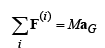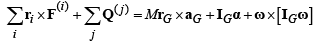For 2D planar motion we can use the simplified formulas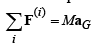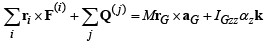6.6.7 Special equations for analyzing bodies that rotate about a stationary point

We often want to predict the motion of a system that rotates about a fixed pivot – a pendulum is a simple example.   These problems can be solved using the equations in 6.6.5 and 6.6.6, but can also be solved using a useful short-cut.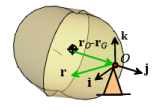• For an object that rotates about a fixed pivot at the origin:
• The total angular momentum (about the origin) is h =IOω
• The total kinetic energy is T = 1/2 ω·I0ω
• The equation of rotational motion is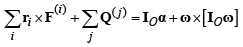Here IO is the mass moment of inertia about O (calculated, eg, using the parallel axis theorem)

For 2D rotation about a fixed point at the origin we can simplify these to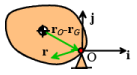• The total angular momentum (about the origin) is h = IOzzω zk
• The total kinetic energy is T=1/2 IOzzωz2
• The equation of rotational motion is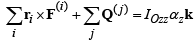Proof: It is straightforward to show these formulas.  Let’s show the two dimensional version of the kinetic energy formulas as an example. For fixed axis rotation, we can use the rigid body formulas to calculate the velocity of the center of mass (O is stationary and at the origin)

vG = ω ×rGzk × rG

The general formula for kinetic energy can therefore be re-written as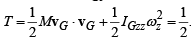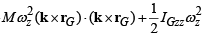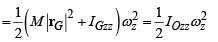The other formulas can be proved with the same method – we simply express the velocity or acceleration of the COM in the general formulas in terms of angular velocity and acceleration, and notice that we can rearrange the result in terms of the mass moment of inertia about O.

Offer running on EduRev: Apply code STAYHOME200 to get INR 200 off on our premium plan EduRev Infinity!

## Introduction to Dynamics and Vibrations- Notes, Videos, MCQs

20 videos|53 docs

,

,

,

,

,

,

,

,

,

,

,

,

,

,

,

,

,

,

,

,

,

;